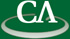Zeros and Poles of diagonal Pade Approximates to functions related to the Riemann Zeta function

Greg Fee, CECM

Wednesday November 22th, 10:30am in IRMACS 10908.

```Abstract:
The Riemann Zeta function is defined by:

Zeta(x) = sum(k^(-x),k=1..infinity).

By using analytic continuation we can extend the definition
of this function from real x>1 to the entire complex plane,
except for a simple pole at x=1. We may remove the pole
by subtracting it from the function or by multiplying
the Zeta function by (x-1), or we can use
Riemann's symmetric Zeta function. First we compute
approximate truncated Taylor series of the above functions
expanded about the points 0 or 1/2 or 1. Next we compute
diagonal Pade approximates to each of the Taylor series.
Then we find the zeros and poles of these Pade approximates.

```